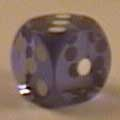<< Home

# Homework

Homeworks will be released weekly. It is mandatory to typeset your solutions using LaTeX. There is a template linked below.Homework 0 (not graded) (LaTeX) (Solution)Homework 1 (LaTeX) (Solution)Homework 2 (LaTeX) (Solution)Homework 3 (LaTeX) (Solution)Homework 4 (LaTeX) (Solution)Homework 5 (LaTeX) (Solution)Midterm (LaTeX) (Solution)Homework 7 (LaTeX) (Solution)Homework 8 (LaTeX) (Solution)Homework 9 (LaTeX) (Solution)Homework 10 (LaTeX) (Solution)Homework 11 (LaTeX) (Solution)Final (LaTeX) ()Template for writing solutions in LaTeX: hw-skel.texBrown CS Latex pageLast Updated: Jan 2020